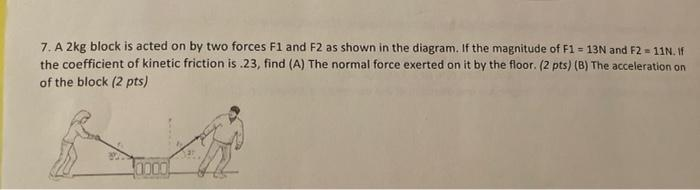# Question Solved1 Answer7. A 2kg block is acted on by two forces F1 and F2 as shown in the diagram. If the magnitude of F1 = 13N and F2 = 11N. the coefficient of kinetic friction is 23, find (A) The normal force exerted on it by the floor. (2 pts) (B) The acceleration on of the block (2 pts) 0000!Transcribed Image Text: 7. A 2kg block is acted on by two forces F1 and F2 as shown in the diagram. If the magnitude of F1 = 13N and F2 = 11N. the coefficient of kinetic friction is 23, find (A) The normal force exerted on it by the floor. (2 pts) (B) The acceleration on of the block (2 pts) 0000!
More
Transcribed Image Text: 7. A 2kg block is acted on by two forces F1 and F2 as shown in the diagram. If the magnitude of F1 = 13N and F2 = 11N. the coefficient of kinetic friction is 23, find (A) The normal force exerted on it by the floor. (2 pts) (B) The acceleration on of the block (2 pts) 0000!